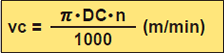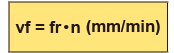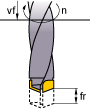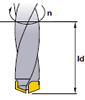## Cutting speed (vc)

Check the item you want to calculate, input values in the two boxes, and then press the Calculate button.*Divide by 1,000 to change to m from mm.

vc (m/min) : Cutting Speed
DC (mm) : Drill Diameter
π (3.14) : Pi
n (min-1) : Main Axis Spindle Speed

n(min-1)
DC(mm)
vc(m/min)

### ProblemWhat is the cutting speed when main axis spindle speed is 1350min-1 and drill diameter is 12mm ?

Substitute π=3.14, DC=12, n=1350 into the formula
vc=π×DC×n÷1000=3.14×12×1350÷1000=50.9m/min
The cutting speed is 50.9m/min.

## Feed of the main spindle (vf)

Check the item you want to calculate, input values in the two boxes, and then press the Calculate button.vf(mm/min)：Feed Speed of the Main Spindle (Z axis)
fr(mm/rev)：Feed per Revolution
n(min-1) ：Main Axis Spindle Speed

fr(mm/rev)
n(min-1)
vf(mm/min)

### ProblemWhat is the spindle feed (vf) when the feed per revolution is 0.2mm/rev and main axis spindle speed is 1350min-1 ?

Substitute fr=0.2,n=1350 into the formula
vf=fr×n=0.2×1350=270mm/min
The spindle feed is 270mm/min.

## Drilling time (Tc)

Input the valuesTc (min) : Drilling Time
n (min-1) : Spindle Speed
ld (mm) : Hole Depth
fr (mm/rev): Feed per Revolution
i : Number of Holes

ld(mm)
i
n(min-1)
fr(mm/rev)
Tc(min)

### ProblemWhat is the drilling time required for drilling a 30mm length hole in alloy steel (JIS SCM440) at a cutting speed of 50m/min and a feed 0.15mm/rev ?